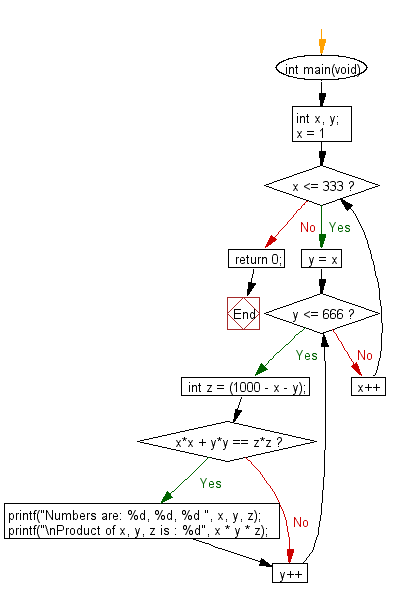﻿ C Program: Find the product xyz - w3resource# C Exercises: Find the product xyz

## C Programming Practice: Exercise-25 with Solution

A Pythagorean triplet is a set of three natural numbers, x < y < z, for which,
x2 + y2 = z2
For example, 32 + 42 = 9 + 16 = 25 = 52.
There exists exactly one Pythagorean triplet for which x +y + z = 1000.
Write a C programming to find the product xyz.

C Code:

``````#include <stdio.h>
#include <stdio.h>

int main(void)
{
int x, y;

for (x = 1; x <= 333; x++) {
for (y = x; y <= 666; y++) {
int z = (1000 - x - y);
if (x*x + y*y == z*z) {
printf("Numbers are: %d, %d, %d ", x, y, z);
printf("\nProduct of x, y, z is : %d", x * y * z);
}
}
}
return 0;
}
``````

Sample Output:

```Numbers are: 200, 375, 425
Product of x, y, z is : 31875000
```

Flowchart:## C Programming Code Editor:

What is the difficulty level of this exercise?

Test your Programming skills with w3resource's quiz.

﻿

## C Programming: Tips of the Day

Maximum value of int:

In C:

```#include <limits.h>
then use
int imin = INT_MIN; // minimum value
int imax = INT_MAX;```

or

```#include <float.h>

float fmin = FLT_MIN;  // minimum positive value
double dmin = DBL_MIN; // minimum positive value

float fmax = FLT_MAX;
double dmax = DBL_MAX;```

Ref : https://bit.ly/3fi8yk9